# Easiest colleges to get into in Oregon

Top 10 colleges in Oregon with the highest acceptance rates
What are the easiest colleges to get into in Oregon? We've got you covered. We've compiled a national college database and have created a list of the easiest colleges to get into in Oregon below. These are the easiest 4 year colleges to get into in Oregon that have the highest acceptance rates, and are more likely to accept low GPAs, GEDs, and everything in between. There are tons of good colleges below that accept more applicants, and we also share their average SAT and ACT scores so that you can see where you're most competitive. Read on to find out more.

## Eastern Oregon University acceptance rate

Eastern Oregon University acceptance rate is 98.4%.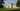The average SAT score for Eastern Oregon University is 1042.

• SAT composite: 1042
• SAT math: 520

The average ACT score for Eastern Oregon University is 20.

## Portland State University acceptance rate

Portland State University acceptance rate is 90%.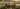The average SAT score for Portland State University is 1119.

• SAT composite: 1119
• SAT math: 550

The average ACT score for Portland State University is 22.

## Willamette University acceptance rate

Willamette University acceptance rate is 83.6%.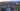The average SAT score for Willamette University is 1230.

• SAT composite: 1230
• SAT math: 620

The average ACT score for Willamette University is 28.

## University of Oregon acceptance rate

University of Oregon acceptance rate is 83.4%.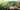The average SAT score for University of Oregon is 1185.

• SAT composite: 1185
• SAT math: 585

The average ACT score for University of Oregon is 25.

## Linfield College acceptance rate

Linfield College acceptance rate is 81%.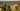The average SAT score for Linfield College is 1110.

• SAT composite: 1110
• SAT math: 550

The average ACT score for Linfield College is 23.

## Oregon State University acceptance rate

Oregon State University acceptance rate is 80.6%.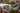The average SAT score for Oregon State University is 1195.

• SAT composite: 1195
• SAT math: 600

The average ACT score for Oregon State University is 25.

## Western Oregon University acceptance rate

Western Oregon University acceptance rate is 80.6%.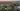The average SAT score for Western Oregon University is 1040.

• SAT composite: 1040
• SAT math: 510

The average ACT score for Western Oregon University is 20.

## Pacific University Oregon acceptance rate

Pacific University Oregon acceptance rate is 79%.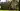The average SAT score for Pacific University Oregon is 1150.

• SAT composite: 1150
• SAT math: 570

The average ACT score for Pacific University Oregon is 24.

## George Fox University acceptance rate

George Fox University acceptance rate is 78.3%.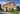The average SAT score for George Fox University is 1150.

• SAT composite: 1150
• SAT math: 560

The average ACT score for George Fox University is 23.

## Southern Oregon University acceptance rate

Southern Oregon University acceptance rate is 78.2%.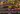The average SAT score for Southern Oregon University is 1100.

• SAT composite: 1100
• SAT math: 530